# RS Aggarwal Solutions for Class 9 Chapter 18: Mean, Median and Mode of Ungrouped Data Exercise 18B

## RS Aggarwal Solutions for Class 9 Maths Exercise 18B PDF

The students can use RS Aggarwal Solutions as a guide to solve the exercise wise problems based on latest CBSE guidelines. The solutions are accurate and explained in a stepwise manner for a better understanding of concepts among students. This exercise mainly contains the formula which can be used in finding means of ungrouped frequency distribution. The students can download PDF and use them as a source of reference for exam preparation. RS Aggarwal Solutions for Class 9 Maths Chapter 18 Mean, Median and Mode of Ungrouped Data Exercise 18B are provided here.

## RS Aggarwal Solutions for Class 9 Chapter 18: Mean, Median and Mode of Ungrouped Data Exercise 18B Download PDF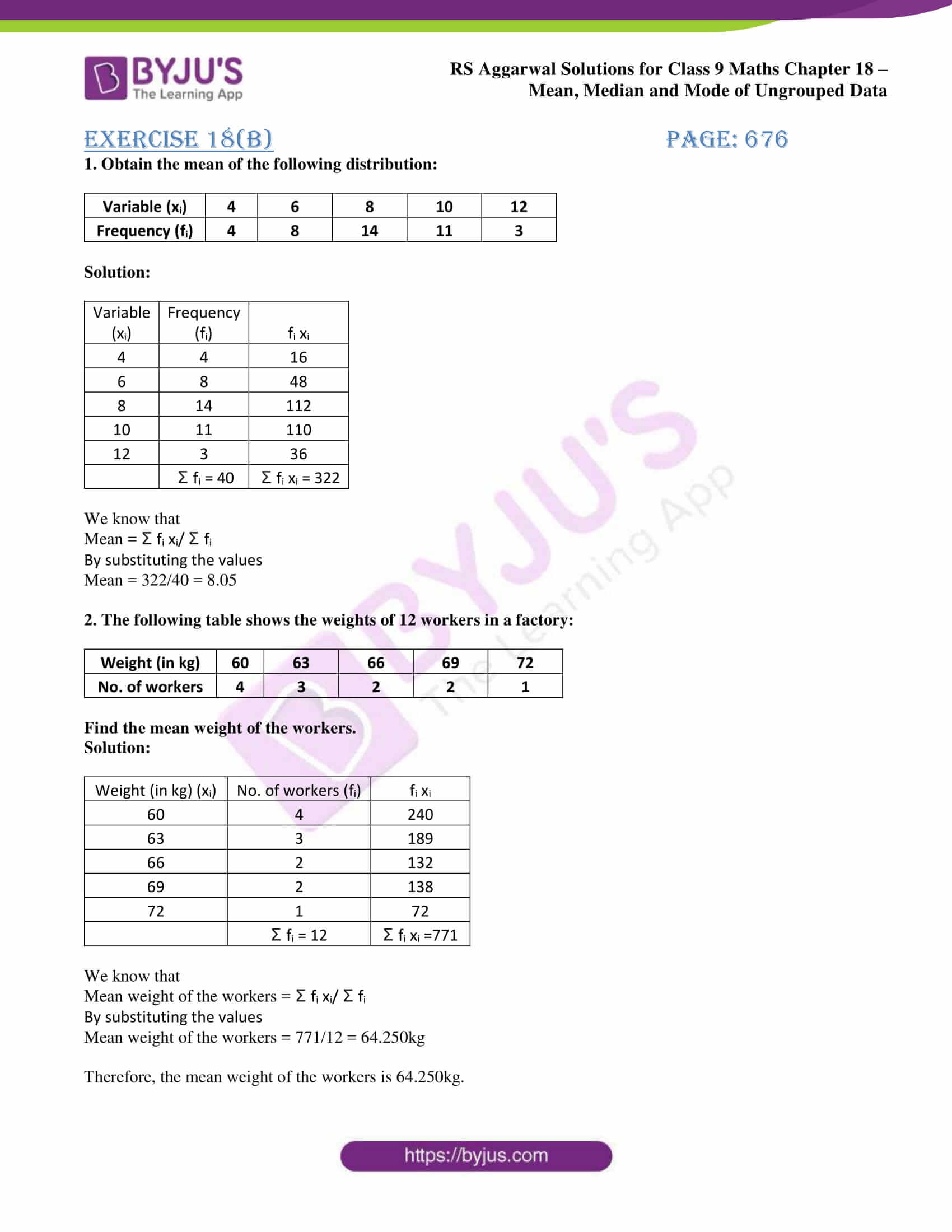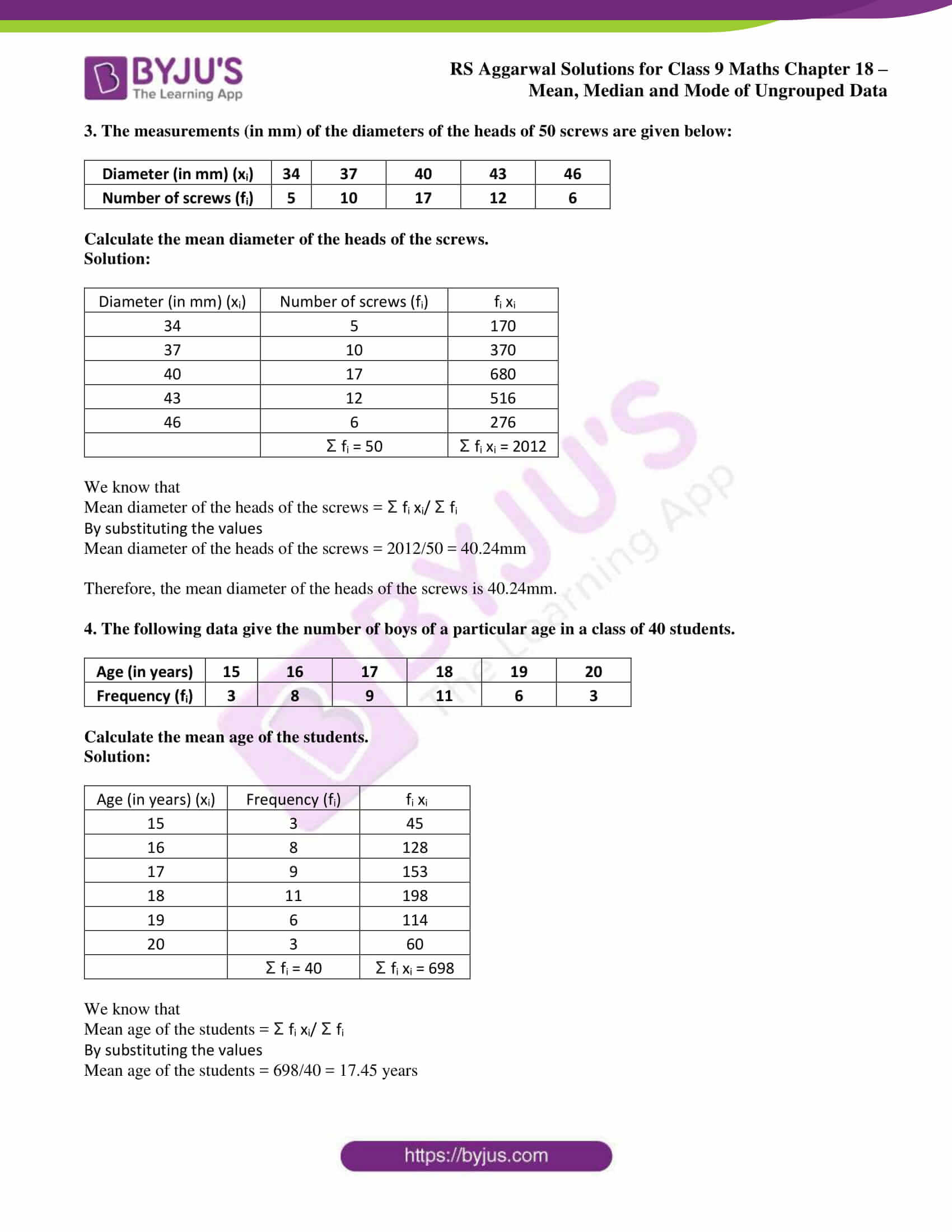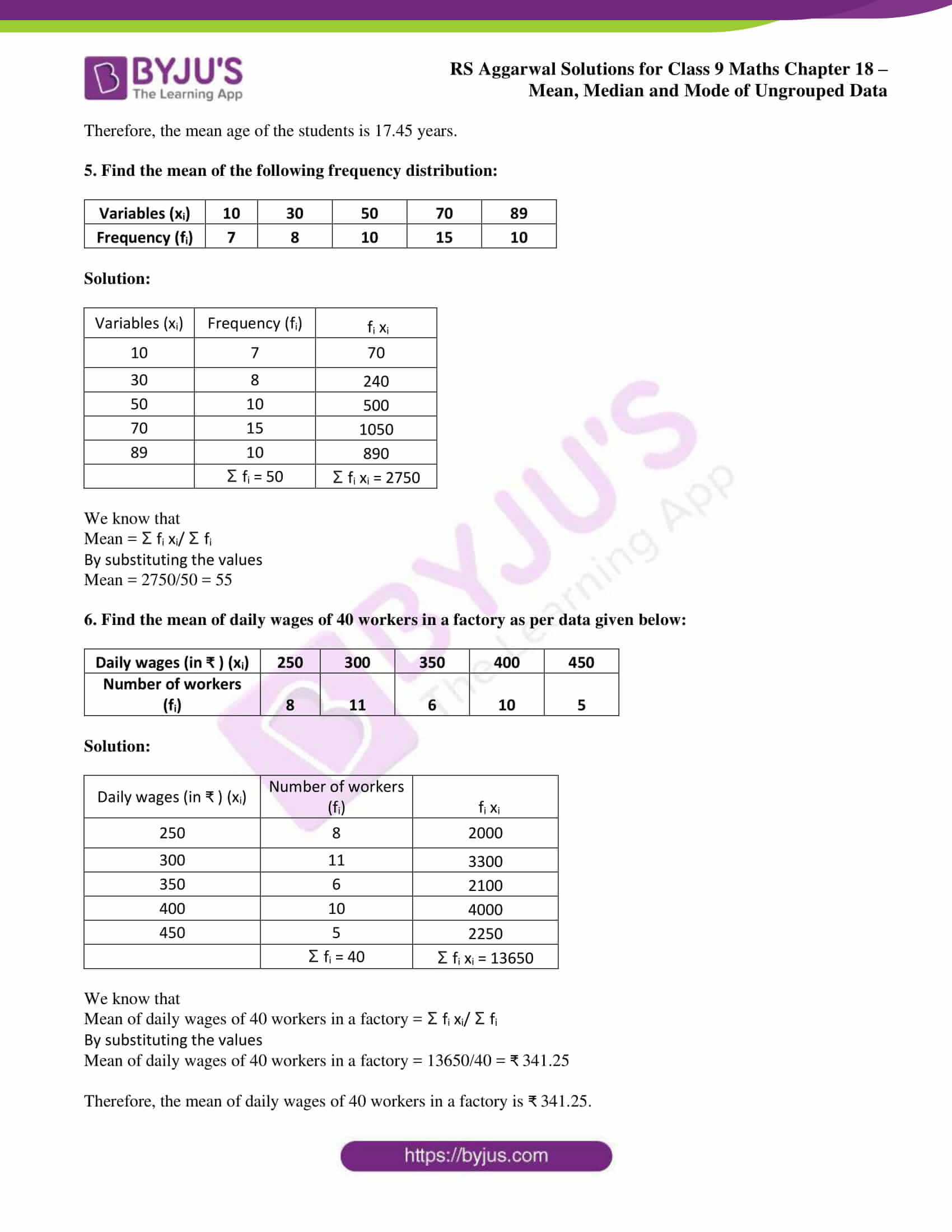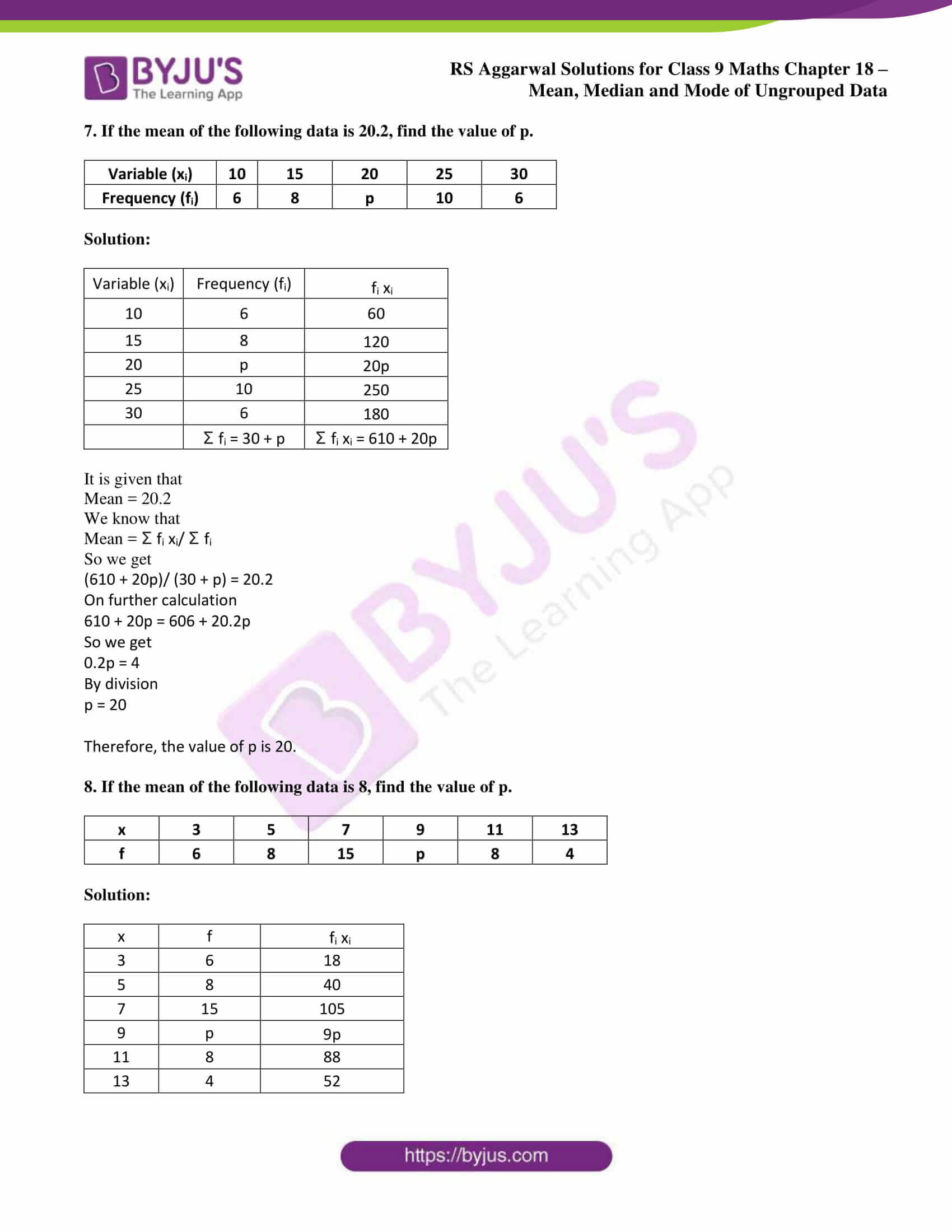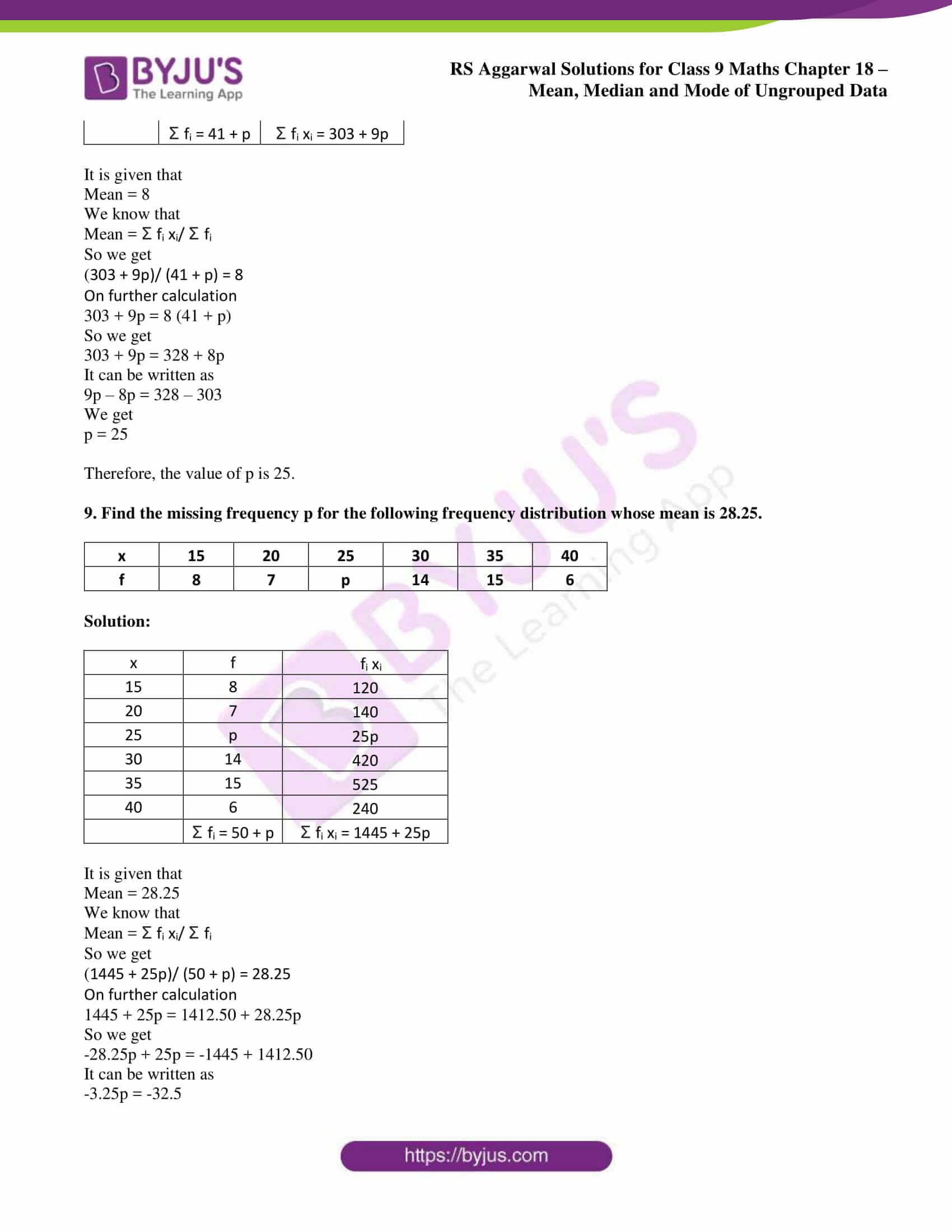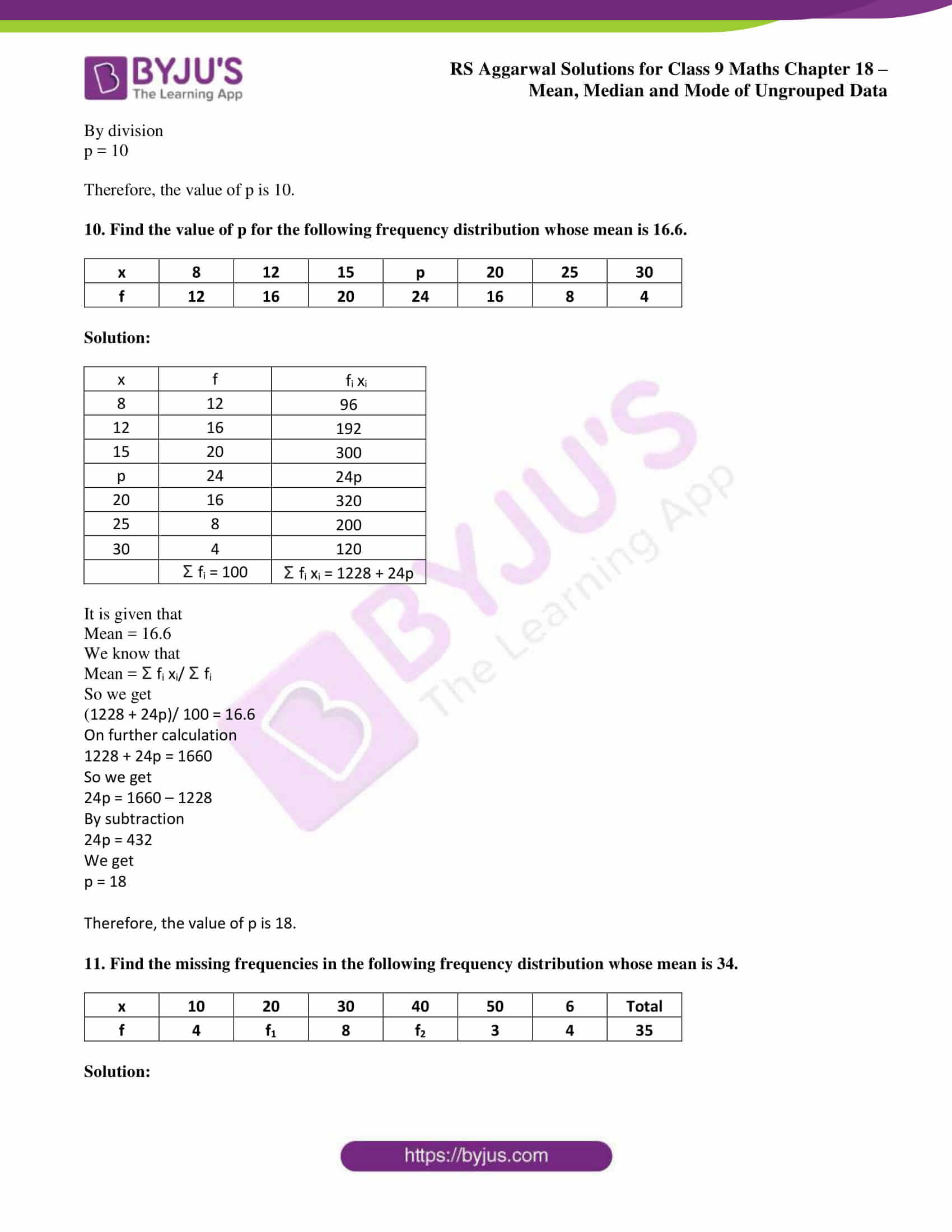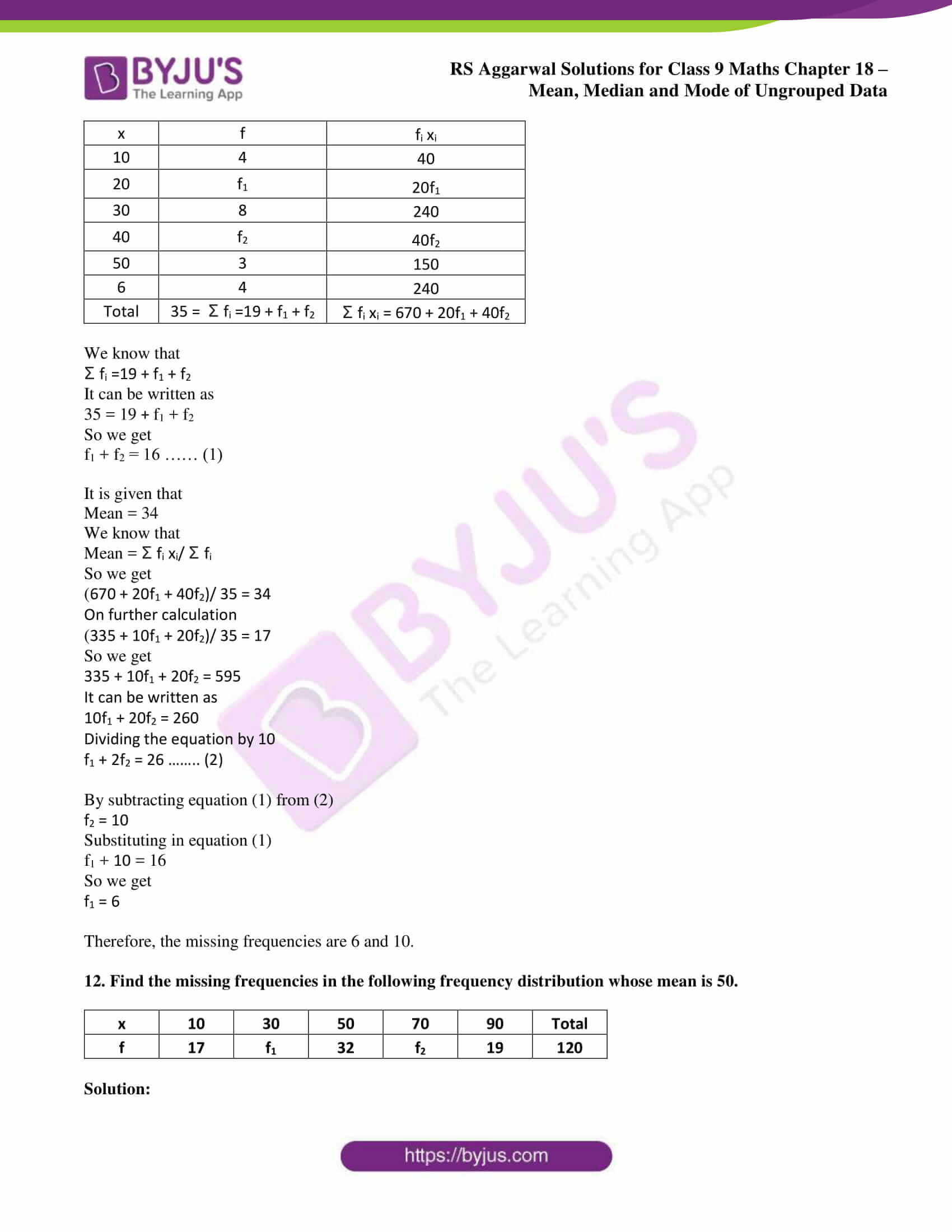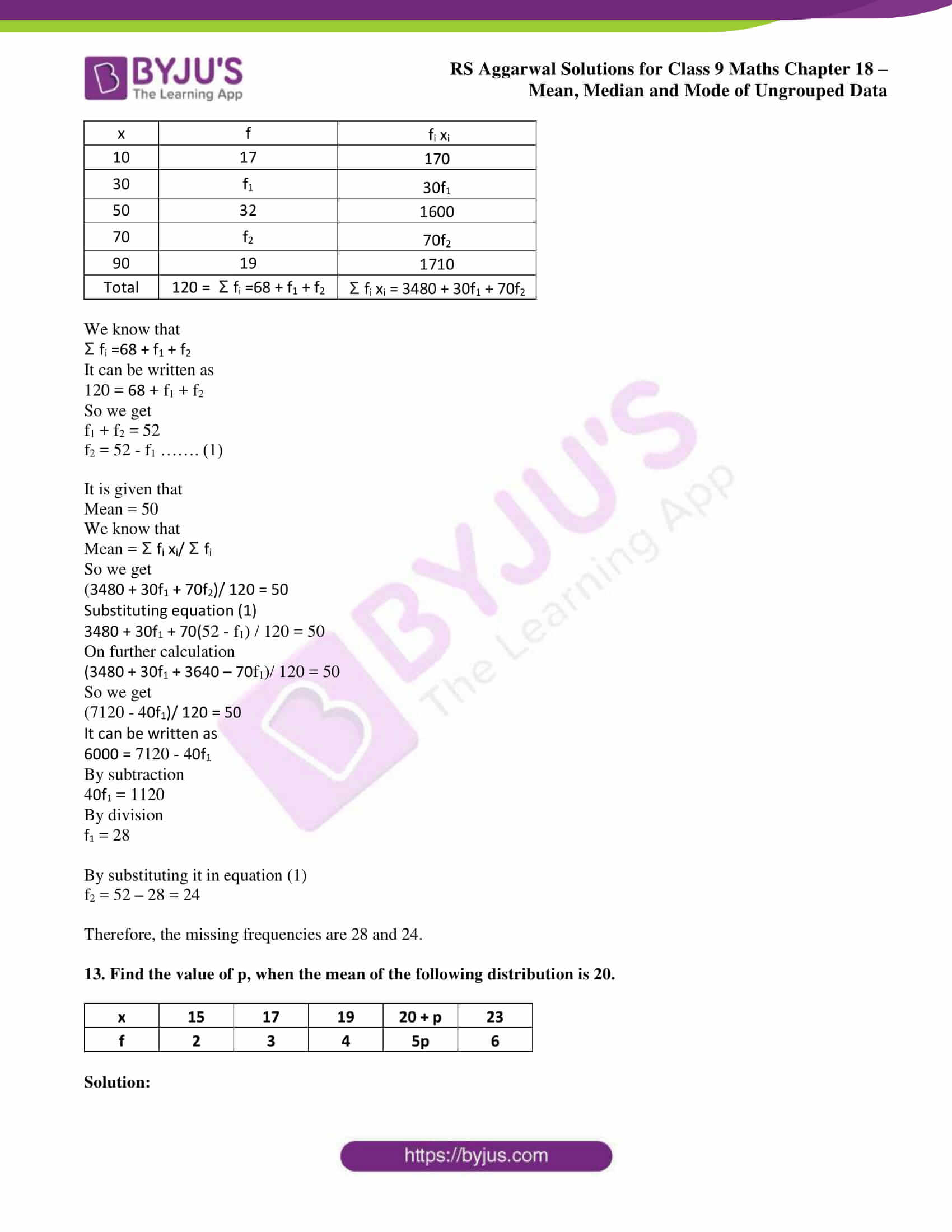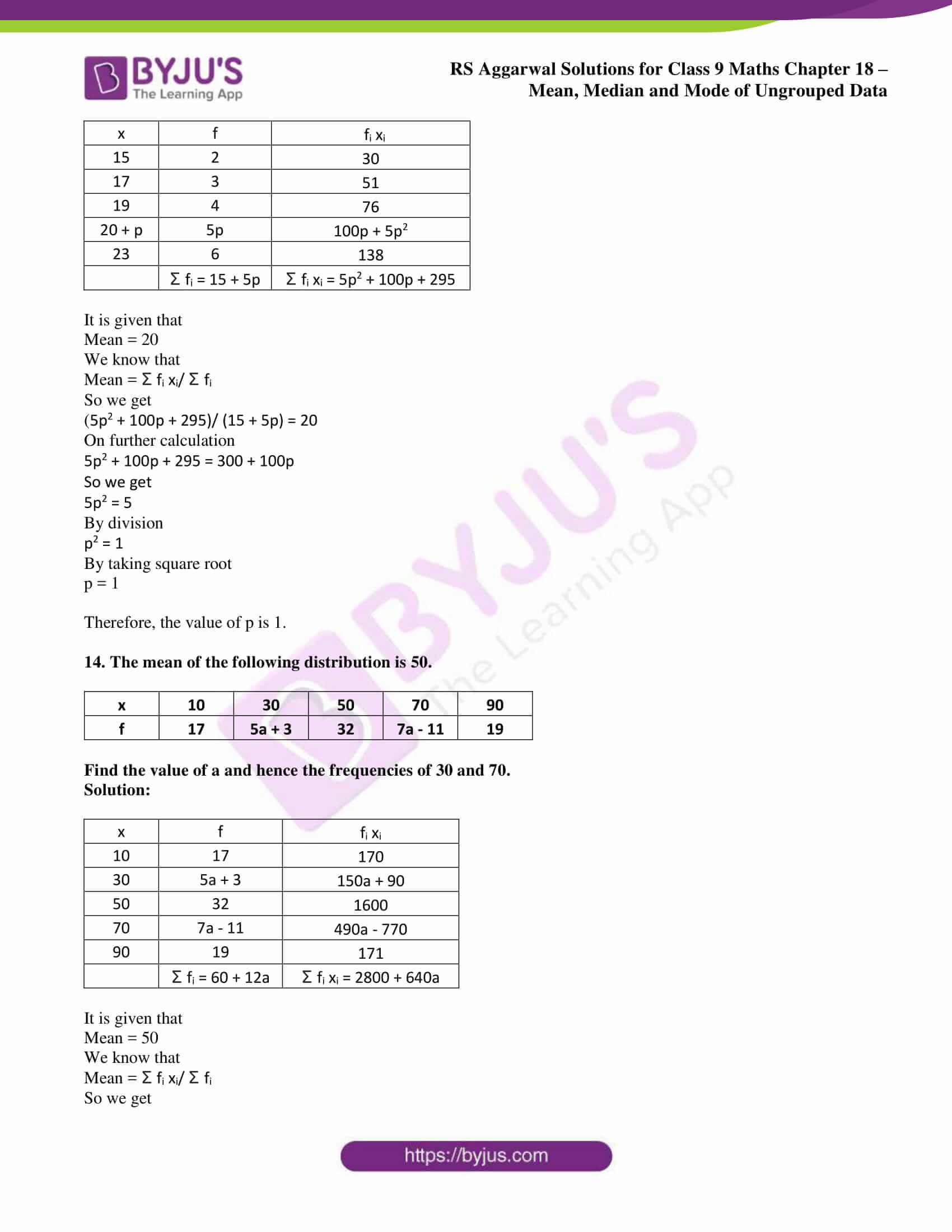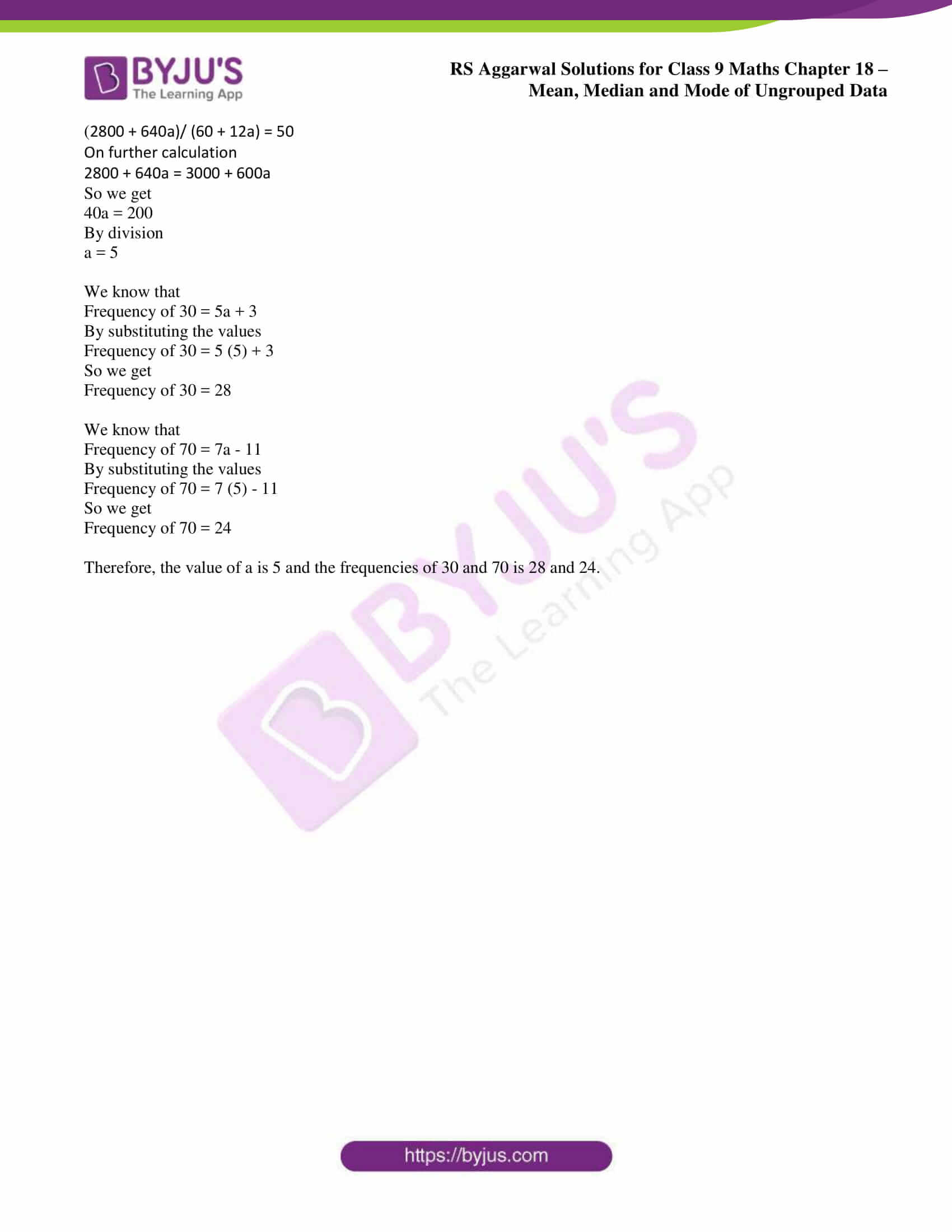## Access RS Aggarwal Solutions for Class 9 Chapter 18: Mean, Median and Mode of Ungrouped Data Exercise 18B

1. Obtain the mean of the following distribution:

 Variable (xi) 4 6 8 10 12 Frequency (fi) 4 8 14 11 3

Solution:

 Variable (xi) Frequency (fi) fi xi 4 4 16 6 8 48 8 14 112 10 11 110 12 3 36 Σ fi = 40 Σ fi xi = 322

We know that

Mean = Σ fi xi/ Σ fi

By substituting the values

Mean = 322/40 = 8.05

2. The following table shows the weights of 12 workers in a factory:

 Weight (in kg) 60 63 66 69 72 No. of workers 4 3 2 2 1

Find the mean weight of the workers.

Solution:

 Weight (in kg) (xi) No. of workers (fi) fi xi 60 4 240 63 3 189 66 2 132 69 2 138 72 1 72 Σ fi = 12 Σ fi xi =771

We know that

Mean weight of the workers = Σ fi xi/ Σ fi

By substituting the values

Mean weight of the workers = 771/12 = 64.250kg

Therefore, the mean weight of the workers is 64.250kg.

3. The measurements (in mm) of the diameters of the heads of 50 screws are given below:

 Diameter (in mm) (xi) 34 37 40 43 46 Number of screws (fi) 5 10 17 12 6

Calculate the mean diameter of the heads of the screws.

Solution:

 Diameter (in mm) (xi) Number of screws (fi) fi xi 34 5 170 37 10 370 40 17 680 43 12 516 46 6 276 Σ fi = 50 Σ fi xi = 2012

We know that

Mean diameter of the heads of the screws = Σ fi xi/ Σ fi

By substituting the values

Mean diameter of the heads of the screws = 2012/50 = 40.24mm

Therefore, the mean diameter of the heads of the screws is 40.24mm.

4. The following data give the number of boys of a particular age in a class of 40 students.

 Age (in years) 15 16 17 18 19 20 Frequency (fi) 3 8 9 11 6 3

Calculate the mean age of the students.

Solution:

 Age (in years) (xi) Frequency (fi) fi xi 15 3 45 16 8 128 17 9 153 18 11 198 19 6 114 20 3 60 Σ fi = 40 Σ fi xi = 698

We know that

Mean age of the students = Σ fi xi/ Σ fi

By substituting the values

Mean age of the students = 698/40 = 17.45 years

Therefore, the mean age of the students is 17.45 years.

5. Find the mean of the following frequency distribution:

 Variables (xi) 10 30 50 70 89 Frequency (fi) 7 8 10 15 10

Solution:

 Variables (xi) Frequency (fi) fi xi 10 7 70 30 8 240 50 10 500 70 15 1050 89 10 890 Σ fi = 50 Σ fi xi = 2750

We know that

Mean = Σ fi xi/ Σ fi

By substituting the values

Mean = 2750/50 = 55

6. Find the mean of daily wages of 40 workers in a factory as per data given below:

 Daily wages (in ₹ ) (xi) 250 300 350 400 450 Number of workers (fi) 8 11 6 10 5

Solution:

 Daily wages (in ₹ ) (xi) Number of workers (fi) fi xi 250 8 2000 300 11 3300 350 6 2100 400 10 4000 450 5 2250 Σ fi = 40 Σ fi xi = 13650

We know that

Mean of daily wages of 40 workers in a factory = Σ fi xi/ Σ fi

By substituting the values

Mean of daily wages of 40 workers in a factory = 13650/40 = ₹ 341.25

Therefore, the mean of daily wages of 40 workers in a factory is ₹ 341.25.

7. If the mean of the following data is 20.2, find the value of p.

 Variable (xi) 10 15 20 25 30 Frequency (fi) 6 8 p 10 6

Solution:

 Variable (xi) Frequency (fi) fi xi 10 6 60 15 8 120 20 p 20p 25 10 250 30 6 180 Σ fi = 30 + p Σ fi xi = 610 + 20p

It is given that

Mean = 20.2

We know that

Mean = Σ fi xi/ Σ fi

So we get

(610 + 20p)/ (30 + p) = 20.2

On further calculation

610 + 20p = 606 + 20.2p

So we get

0.2p = 4

By division

p = 20

Therefore, the value of p is 20.

8. If the mean of the following data is 8, find the value of p.

 x 3 5 7 9 11 13 f 6 8 15 p 8 4

Solution:

 x f fi xi 3 6 18 5 8 40 7 15 105 9 p 9p 11 8 88 13 4 52 Σ fi = 41 + p Σ fi xi = 303 + 9p

It is given that

Mean = 8

We know that

Mean = Σ fi xi/ Σ fi

So we get

(303 + 9p)/ (41 + p) = 8

On further calculation

303 + 9p = 8 (41 + p)

So we get

303 + 9p = 328 + 8p

It can be written as

9p – 8p = 328 – 303

We get

p = 25

Therefore, the value of p is 25.

9. Find the missing frequency p for the following frequency distribution whose mean is 28.25.

 x 15 20 25 30 35 40 f 8 7 p 14 15 6

Solution:

 x f fi xi 15 8 120 20 7 140 25 p 25p 30 14 420 35 15 525 40 6 240 Σ fi = 50 + p Σ fi xi = 1445 + 25p

It is given that

Mean = 28.25

We know that

Mean = Σ fi xi/ Σ fi

So we get

(1445 + 25p)/ (50 + p) = 28.25

On further calculation

1445 + 25p = 1412.50 + 28.25p

So we get

-28.25p + 25p = -1445 + 1412.50

It can be written as

-3.25p = -32.5

By division

p = 10

Therefore, the value of p is 10.

10. Find the value of p for the following frequency distribution whose mean is 16.6.

 x 8 12 15 p 20 25 30 f 12 16 20 24 16 8 4

Solution:

 x f fi xi 8 12 96 12 16 192 15 20 300 p 24 24p 20 16 320 25 8 200 30 4 120 Σ fi = 100 Σ fi xi = 1228 + 24p

It is given that

Mean = 16.6

We know that

Mean = Σ fi xi/ Σ fi

So we get

(1228 + 24p)/ 100 = 16.6

On further calculation

1228 + 24p = 1660

So we get

24p = 1660 – 1228

By subtraction

24p = 432

We get

p = 18

Therefore, the value of p is 18.

11. Find the missing frequencies in the following frequency distribution whose mean is 34.

 x 10 20 30 40 50 6 Total f 4 f1 8 f2 3 4 35

Solution:

 x f fi xi 10 4 40 20 f1 20f1 30 8 240 40 f2 40f2 50 3 150 6 4 240 Total 35 = Σ fi =19 + f1 + f2 Σ fi xi = 670 + 20f1 + 40f2

We know that

Σ fi =19 + f1 + f2

It can be written as

35 = 19 + f1 + f2

So we get

f1 + f2 = 16 …… (1)

It is given that

Mean = 34

We know that

Mean = Σ fi xi/ Σ fi

So we get

(670 + 20f1 + 40f2)/ 35 = 34

On further calculation

(335 + 10f1 + 20f2)/ 35 = 17

So we get

335 + 10f1 + 20f2 = 595

It can be written as

10f1 + 20f2 = 260

Dividing the equation by 10

f1 + 2f2 = 26 …….. (2)

By subtracting equation (1) from (2)

f2 = 10

Substituting in equation (1)

f1 + 10 = 16

So we get

f1 = 6

Therefore, the missing frequencies are 6 and 10.

12. Find the missing frequencies in the following frequency distribution whose mean is 50.

 x 10 30 50 70 90 Total f 17 f1 32 f2 19 120

Solution:

 x f fi xi 10 17 170 30 f1 30f1 50 32 1600 70 f2 70f2 90 19 1710 Total 120 = Σ fi =68 + f1 + f2 Σ fi xi = 3480 + 30f1 + 70f2

We know that

Σ fi =68 + f1 + f2

It can be written as

120 = 68 + f1 + f2

So we get

f1 + f2 = 52

f2 = 52 – f1 ……. (1)

It is given that

Mean = 50

We know that

Mean = Σ fi xi/ Σ fi

So we get

(3480 + 30f1 + 70f2)/ 120 = 50

Substituting equation (1)

3480 + 30f1 + 70(52 – f1) / 120 = 50

On further calculation

(3480 + 30f1 + 3640 – 70f1)/ 120 = 50

So we get

(7120 – 40f1)/ 120 = 50

It can be written as

6000 = 7120 – 40f1

By subtraction

40f1 = 1120

By division

f1 = 28

By substituting it in equation (1)

f2 = 52 – 28 = 24

Therefore, the missing frequencies are 28 and 24.

13. Find the value of p, when the mean of the following distribution is 20.

 x 15 17 19 20 + p 23 f 2 3 4 5p 6

Solution:

 x f fi xi 15 2 30 17 3 51 19 4 76 20 + p 5p 100p + 5p2 23 6 138 Σ fi = 15 + 5p Σ fi xi = 5p2 + 100p + 295

It is given that

Mean = 20

We know that

Mean = Σ fi xi/ Σ fi

So we get

(5p2 + 100p + 295)/ (15 + 5p) = 20

On further calculation

5p2 + 100p + 295 = 300 + 100p

So we get

5p2 = 5

By division

p2 = 1

By taking square root

p = 1

Therefore, the value of p is 1.

14. The mean of the following distribution is 50.

 x 10 30 50 70 90 f 17 5a + 3 32 7a – 11 19

Find the value of a and hence the frequencies of 30 and 70.

Solution:

 x f fi xi 10 17 170 30 5a + 3 150a + 90 50 32 1600 70 7a – 11 490a – 770 90 19 171 Σ fi = 60 + 12a Σ fi xi = 2800 + 640a

It is given that

Mean = 50

We know that

Mean = Σ fi xi/ Σ fi

So we get

(2800 + 640a)/ (60 + 12a) = 50

On further calculation

2800 + 640a = 3000 + 600a

So we get

40a = 200

By division

a = 5

We know that

Frequency of 30 = 5a + 3

By substituting the values

Frequency of 30 = 5 (5) + 3

So we get

Frequency of 30 = 28

We know that

Frequency of 70 = 7a – 11

By substituting the values

Frequency of 70 = 7 (5) – 11

So we get

Frequency of 70 = 24

Therefore, the value of a is 5 and the frequencies of 30 and 70 is 28 and 24.

### s 9 Maths Chapter 18: Mean, Median and Mode of Ungrouped Data

Exercise 18A Solutions 31 Questions

Exercise 18C Solutions 10 Questions

Exercise 18D Solutions 8 Questions

### RS Aggarwal Solutions Class 9 Maths Chapter 18 – Mean, Median and Mode of Ungrouped Data Exercise 18B

RS Aggarwal Solutions Class 9 Maths Chapter 18 Mean, Median and Mode of Ungrouped Data Exercise 18B explains the method of finding means of an ungrouped frequency distribution otherwise known as the direct method.

### Key features of RS Aggarwal Solutions for Class 9 Maths Chapter 18: Mean, Median and Mode of Ungrouped Data Exercise 18B

• The students can use PDF of solutions while solving the RS Aggarwal textbook to find the other methods of answering problems.
• Time management abilities are improved among students, which is one of the important aspects from the exam point of view.
• The answers are explained in simple language which match the understanding capacity of students.
• The subject experts prepare answers based on CBSE guidelines to help students obtain better academic scores.